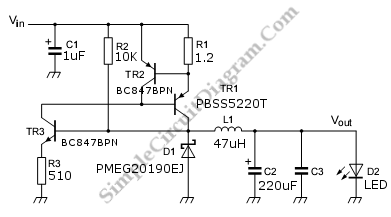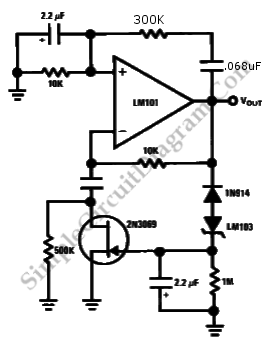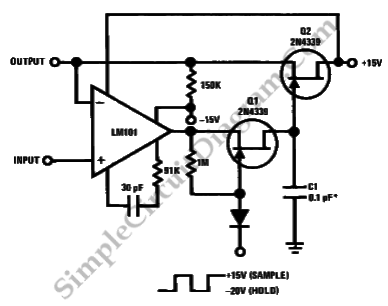## Discrete High Current Switch Mode LED DriverThis is a discrete high current switch mode LED driver circuit. The basics of this circuit is a buck-converter principle. The efficiency of this circuit is 80 to 90 %. This circuit can be applied on automotive lighting applications. The advantages of this circuit are low component and low cost. Here is the circuit: The voltage input range of this […]

## Wien Bridge Sine Wave OscillatorGetting the right amplifier loop gain is the major problem in producing a low distortion constant amplitude sine wave. However, we can solve this problem with Wien bridge sine wave oscillator. Here is the circuit : This circuit uses the 2N3069 JFET as a voltage variable resistor in the amplifier feedback loop. The voltage reference for the peak sine wave […]